﻿ 机电集成电磁蜗杆传动的磁场分析 Magnetic Field Analysis of Electromechanical Integration Electromagnetic Worm Drive

Mechanical Engineering and Technology
Vol. 08  No. 03 ( 2019 ), Article ID: 31061 , 9 pages
10.12677/MET.2019.83034

Magnetic Field Analysis of Electromechanical Integration Electromagnetic Worm Drive

Xuemei Guan

College of Vehicles and Energy, Yanshan University, Qinhuangdao Hebei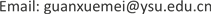Received: June 6th, 2019; accepted: June 21st, 2019; published: June 28th, 2019ABSTRACT

In this paper, the electromagnetic force calculation model of electromechanical integrated electromagnetic worm drive is established, and the electromagnetic force calculation formula in air gap is derived by using the electromagnetic theory, and the parameter change curve related to electromagnetic force is obtained. The output torque of the system is calculated by using ampere loop method and magnetic circuit method. The formula for calculating the magnetic field intensity of different magnetic poles under uniform magnetization is derived, and a two-dimensional magnetic field model is established. The results show that the air gap between worm gear and worm is as small as possible. When the system is designed, more permanent magnets are used. Taking the result of analysis into consideration, the permanent magnet adopts radial magnetization mode.

Keywords:Electromechanical Integration, Electromagnetic Worm Drive, Magnetic Field Analysis, Electromagnetic Force, Finite Element Analysis1. 引言

2. 电磁力计算模型

3. 空气隙中的电磁力

1) 在电磁蜗杆、蜗轮和气隙中磁场是分段均匀的。

2) 铁心的磁感应强度不能高于其饱和磁感应强度，即处于不饱和状态。

3) 假设磁通全部流过铁心，不考虑漏磁和磁滞的影响。

4) 蜗杆上的线圈和电源之间没有电能交换。

5) 忽略铁芯磁阻误差。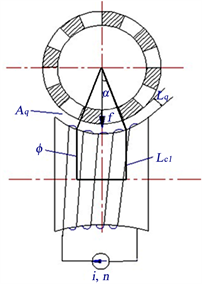Figure 1. Equivalent magnetic loop analysis

$f=\frac{{B}_{0}^{2}{A}_{q}}{{\mu }_{0}}$ (1)

$Ni=\oint H\text{d}s={L}_{cl}{H}_{2}+2{L}_{q}{H}_{0}$ (2)

${f}_{i}={\left(\frac{Ni}{\frac{{L}_{cl}}{{\mu }_{}}+\frac{2{L}_{q}}{{\mu }_{0}}}\right)}^{2}\frac{{A}_{q}}{{\mu }_{0}}={\left(\frac{Ni}{\frac{{L}_{cl}}{{\mu }_{2r}}+2{L}_{q}}\right)}^{2}{\mu }_{0}{A}_{q}$ (3)

${f}_{i}={\left(\frac{Ni}{2{L}_{q}}\right)}^{2}{\mu }_{0}{A}_{q}=\frac{{i}^{2}}{{L}^{2}{}_{q}}\frac{{N}^{2}{\mu }_{0}{A}_{q}}{4}=k\frac{{i}^{2}}{{L}^{2}{}_{q}}$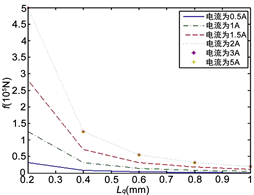(a) N = 4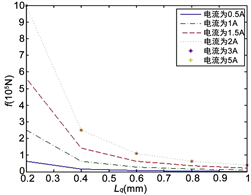(b) N = 6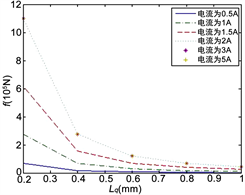(c) N = 8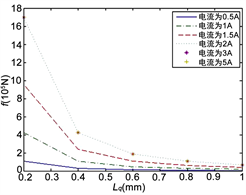(d) N = 10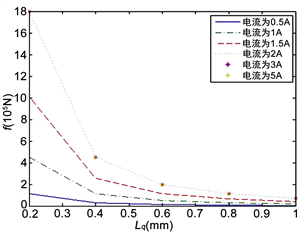(e) N = 12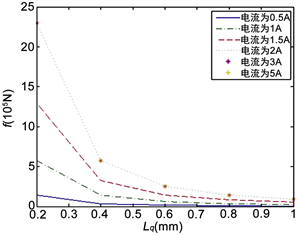(f) N = 14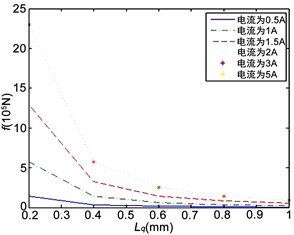(g) N = 16(h) N = 18(i) N = 20

Figure 2. The electromagnetic force distribution of different magnetic poles

1) 不同磁极的电磁力分布图形大致一样，当蜗轮磁极数增加时，其啮合磁极数也增加，因而电磁力大小也随着增大。

2) 影响电磁力大小的主要有蜗杆线圈的通电电流和空气隙。电磁力的大小主要受空气隙的影响比较大，蜗杆线圈的通电电流对电磁力的影响在其取值小的时候比较明显，取值大时则对电磁力影响不大。

3) 当空气隙由0.2 mm增加到0.4 mm时，电磁力迅速减小，当空气隙由0.4 mm增加到1 mm时，电磁力缓慢减小。

4) 当通电电流由0.5 A增加到1.5 A时，电磁力随着电流的增加而迅速增加，当通电电流由1.5 A增加到5 A时，电磁力增加的非常缓慢。

4. 系统输出力矩

${T}_{n}={f}_{q}R$

5. 电磁蜗杆传动二维有限元分析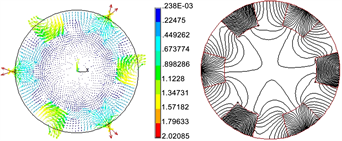(a) N = 6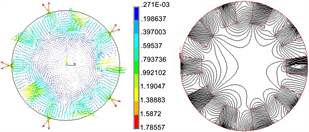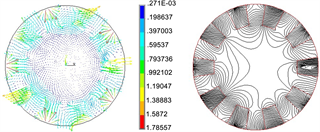(b) N = 10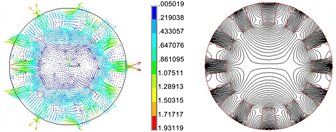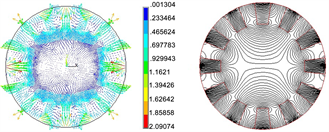(c) N = 12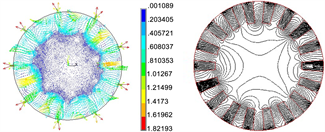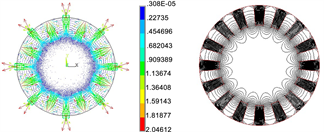(d) N = 16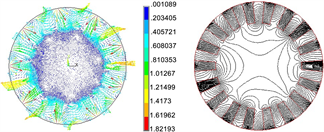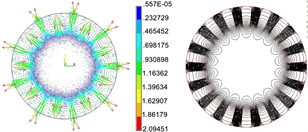(e) N = 18(磁感应强度单位：T，磁力线根数：50)

Figure 3. The line of magnetic force and magnetic induction distribution of different poles (Radial and tangential crisscross magnetization)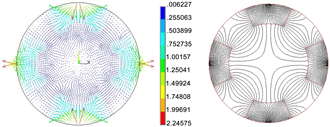(a) N = 4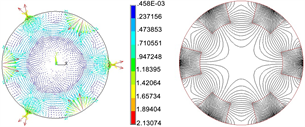(b) N = 6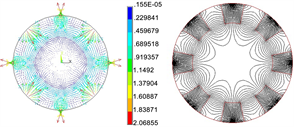(c) N = 8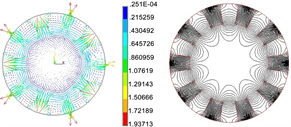(d) N = 10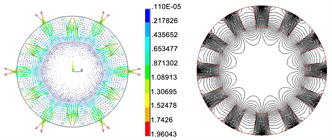(e) N = 12(f) N = 14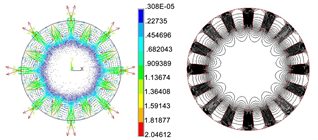(g) N = 16(h) N = 18

Figure 4. The line of magnetic force and magnetic induction distribution of different poles (Radial magnetization)

1) 从磁力线分布图上可以得出：磁通流线密的地方表示该处磁感应强度大，流线稀疏的地方表示该处磁感应强度弱，并且和磁感应强度分布规律相对应，磁通流线主要集中分布于磁体附近，并且以永磁体径向中心线为对称分布，在中心线最外侧处磁通流线最密。

2) 从磁感应强度分布图中可以看到：永磁体附近的磁感应强度数值大约在0.2 T~1.5 T左右。其中有黄线和红线的地方，表明该处的场强较强，而出现场强较强的地方主要在两块永磁体交界处，这是由于在角点处没有做钝化处理的结果。磁感应强度在稍远于永磁体附近的区域中是蓝色区域，表明场强较弱。在磁体外侧边缘的区域中磁感应强度大多为绿色和黄色，表明该处的场强较强，这也是电磁蜗杆传动的工作区，为了避免磁场强度衰减过快，蜗轮与蜗杆之间气隙尽量小。

3) 通过不同充磁方式情况下磁感应强度最高值和最低值比较来看：径向切向交错充磁与径向充磁的最高值相差不多，总体上是径向充磁数值高点；径向切向交错充磁时，不同极数其最小磁感应强度值相差很多，最少相差几十倍，最多相差几万倍，因此在比较图上舍去了最大数值(N = 4、N = 6时)，径向充磁的最小值比径向切向交错充磁最小值小的很多。

4) 从漏磁区的磁力线分布可以看出：随着永磁体个数的增加，漏磁区的范围减小，因此在系统设计时蜗轮尽可能采用较多的永磁体数，较多的永磁体数也有利于增加蜗轮和蜗杆的啮合齿数，从而增加力矩输出。

6. 结论

1) 为了提高永磁体的利用效率，必须尽量减小气隙，气隙控制在1 mm范围为好。随着磁极数增加，磁漏区范围也越小，所以实际应用中要尽可能采用数量多的磁极。随着磁极数增加，每个磁极的磁通密度也增加，因而传递的力矩也越大。

2) 为避免磁场强度衰减过快，蜗轮与蜗杆之间气隙应该尽量小，气隙控制在1 mm范围为好。

3) 电磁蜗杆传动工作区域是永磁体外侧，内侧不是工作区，不用考虑最小值取值的蓝色区域，综合考虑永磁体采用径向充磁方式。

4) 系统设计时蜗轮尽可能采用较多的永磁体数。

Magnetic Field Analysis of Electromechanical Integration Electromagnetic Worm Drive[J]. 机械工程与技术, 2019, 08(03): 268-276. https://doi.org/10.12677/MET.2019.83034

1. 1. 孙家广. 对我国制造业信息化的思考[J]. 中国制造业信息化, 2010(10): 10-12.

2. 2. 郭力, 李波, 朱均, 等. 21世纪机械科学的展望[J]. 精密制造与自动化, 2001, 145(1): 7-9.

3. 3. 陈虹, 李赠铨. 中国先进制造业国际竞争力的实证分析[J]. 统计与决策, 2019, 523(7): 154-157.

4. 4. 刘冰. 浅谈光机电一体化技术的发展方向[J]. 论坛, 2010(36): 133-134.

5. 5. 关雪梅, 许立忠. 机电集成电磁蜗杆传动的运动机理分析[J]. 机械设计, 2016, 33(6): 6-9.

6. 6. 蔡毅, 许立忠. 机电集成传动的啮合原理研究[J]. 机械设计, 1998, 15(4): 42-43.

7. 7. 米春亭, 陆鹤庆. 稀土永磁同步电动机磁路计算方法[J]. 微电机, 1992, 25(3): 12-17.

8. 8. 许金, 聂世雄, 马伟明. 无槽双边长定子直线感应电动机磁路计算方法[J]. 中国电机工程学报, 2016, 36(10): 2793-2798.

9. 9. 田杰, 赵韩. 小型及微型稀土永磁传动设计初探[J]. 机械传动, 1998, 22(2): 22-24.

10. 10. 田杰. 稀土永磁齿轮的设计理论与实验研究[D]: [博士学位论文]. 合肥: 合肥工业大学, 1999: 38-47.

11. 11. 黄允凯, 朱建国, 胡虔生. 采用软磁复合材料设计高速爪极式永磁电机[J]. 电机与控制应用, 2007, 34(12): 6-13.